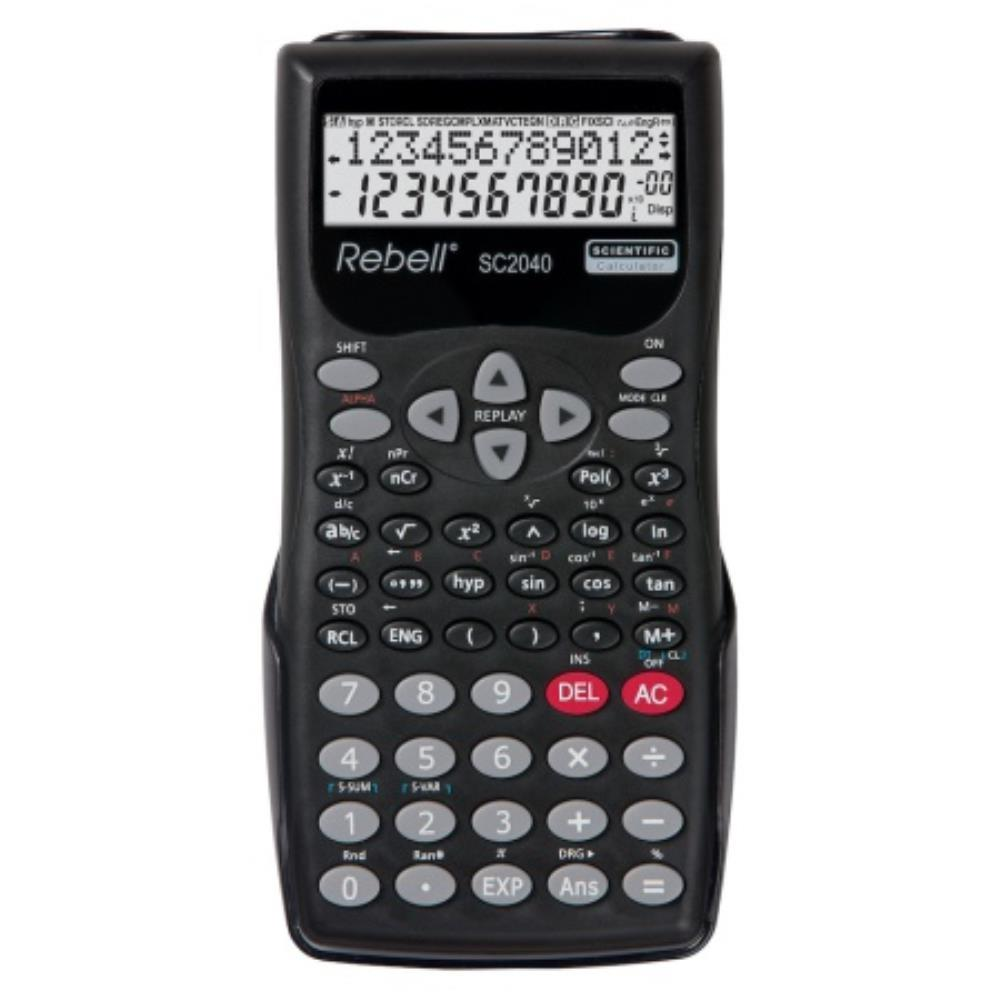# Rebell SC2040 Standard 2-Line GCSE Calculator - Black

Code: CAL1084 N1-1

Scientific calculator with 240 mathematical functions and 2-line display, ideal for high school students. Robust design with hard protective case and plastic keys.

• 240 integrated functio...

£4.58 EACH
List PriceScientific calculator with 240 mathematical functions and 2-line display, ideal for high school students. Robust design with hard protective case and plastic keys.

• 240 integrated functions
• 9 variable memories
• Trigonometric and hyperbolic functions
• Polar and rectangular coordinates
• Calculates with fractions
• Permutation, combinational logic
• Two-dimensional statistics
• Display: 12 digit
• Power supply: battery
• Weight: 117g
• Dimensions: 155 x 70 x 18mm